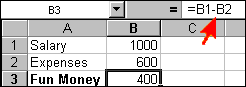## Redirected from: worksheet program

Software that simulates a paper spreadsheet (worksheet), in which columns of numbers are summed for budgets and plans. It appears on screen as a matrix of rows and columns, the intersections of which are called "cells." The cells are filled with (1) labels, (2) numeric values or (3) formulas.

Labels are descriptive text such as "Rent" and "Gross Sales." Values are the actual numeric data, and formulas command the spreadsheet to do the calculations; for example, SUM CELLS A5 TO A10.Labels, Values and Formulas
In this Microsoft Excel example, the labels are in cells A1, A2 and A3, and numeric values are in B1 and B2. The formula in B3 is "subtract B2 from B1." In Excel, typing the equals sign starts the formula creation.

The Formulas
Formulas are the spreadsheet's magic, and they are easy to create. You click a cell and then press the key (+, -, etc.) of the arithmetic operation that affects it. For example, the creation of a formula might be "the contents of this cell PLUS the contents of this cell DIVIDED BY the contents of this cell."

The Ripple Effect
After numbers are added or changed, the formulas recalculate the data automatically or with the press of a key. Since the contents of any cell can be calculated with or copied to any other cell, a total of one column can be used as a detail item in another column. For example, the total from a column of expense items can be carried over to a summary column showing all expenses. If the contents of a cell in the detail column changes, its column total changes, which is then copied to the summary column, and the summary total changes.

What If?
The ripple effect lets you create a plan, plug in different assumptions and immediately see the impact on the bottom line. This "what if?" capability makes the spreadsheet indispensable for budgets, plans and other equation-based tasks.

It Started with VisiCalc
One of the major forces behind the personal computer revolution in the 1980s, the spreadsheet originated with VisiCalc in 1978 for the Apple II, followed by SuperCalc, Multiplan, Lotus 1-2-3 and others. See VisiCalc, OLAP, analytical database engine and XL abc's.